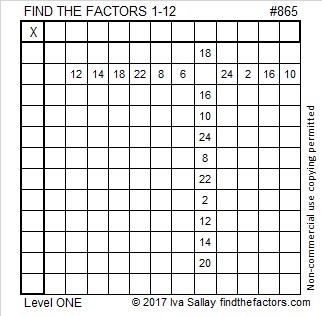# 865 and Level 1Print the puzzles or type the solution on this excel file: 12 factors 864-874

865 is the sum of two squares two different ways:

• 28² + 9² = 865
• 24² + 17² = 865

865 is the hypotenuse of four Pythagorean triples, two of which are primitives:

• 260-825-865, which is 5 times (52-165-173)
• 287-816-865, which is 24² – 17², 2(24)(17), 24² + 17²
• 504-703-865 which is 2(28)(9), 28² – 9², 28² – 9²
• 519-692-865, which is (3-4-5) times 173

You could see 865’s factors in two of those Pythagorean triples, and here they are again:

• 865 is a composite number.
• Prime factorization: 865 = 5 × 173
• The exponents in the prime factorization are 1 and 1. Adding one to each and multiplying we get (1 + 1)(1 + 1) = 2 × 2 = 4. Therefore 865 has exactly 4 factors.
• Factors of 865: 1, 5, 173, 865
• Factor pairs: 865 = 1 × 865 or 5 × 173
• 865 has no square factors that allow its square root to be simplified. √865 ≈ 29.41088
1.davescarthin
•ivasallay